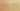# RecursiveFunctionNeedsIfElse

Misconception:

If a function does not have an if-else statement, then it cannot have a base case and a recursive case, and thus it cannot properly implement a recursive computation that can terminate at some point (by hitting the base case).Incorrect

A recursive function needs to contain an if-else statement

Correct

A recursive function does not necessarily need to contain an if-else statement

## CorrectionHere is what's right.

Sometimes a simple `if` statement, without an `else`, can be enough.

This kind of single-branch if statement in a recursive function is common when the computation involves side effects (is not a pure function) and the base case means “no action”.

Another situation where no if-else statement is needed is if the recursive call is polymorphic, and one of the possible call targets corresponds to the base case:

``````class List:
...

class Nil(List):
def contains(self, value: int) -> bool:
return False

class Cons(List):
def __init__(self, value: int, tail: List):
self.value = value
self.tail = tail

def contains(self, value: int) -> bool:
return self.value == value or self.tail.contains(value)``````

The recursive call site represents a dynamic dispatch, either to `Nil.contains()` (base case) or to `Cons.contains()` (recursive case).

## OriginWhere could this misconception come from?

This misconception might just be students inappropriately refining the “mantra” that you need a base case and a recursive case i.e, “you need an if branch and an else branch”.

However, it could also be that this misconception is more about if statements than about recursion. For example, if students previously learned functional programming with a textbook like HtDP, then they are used to pure functions built from expressions with `if` or `cond`. And such conditional expressions have to explicitly cover all cases (e.g., there must always be an else case in the if).

## ValueHow can you build on this misconception?

Students who hold this misconception seem to have internalized that one does need a base case and a recursive case to properly implement a finite recursive computation.

This is valuable!

Python